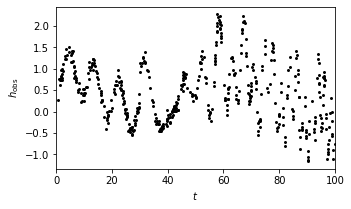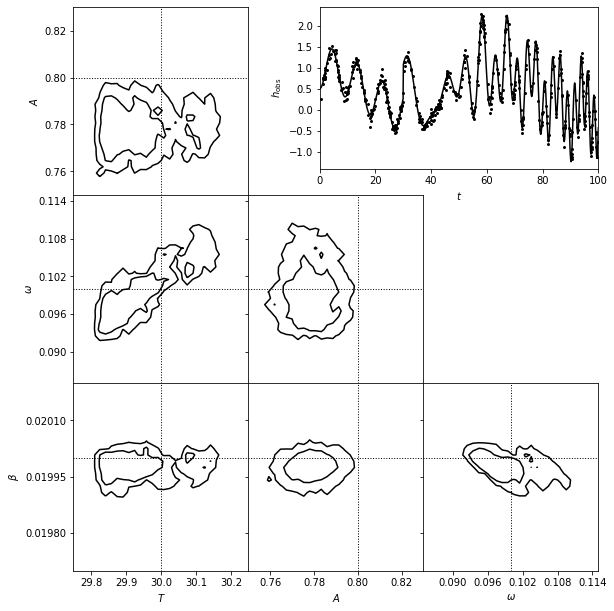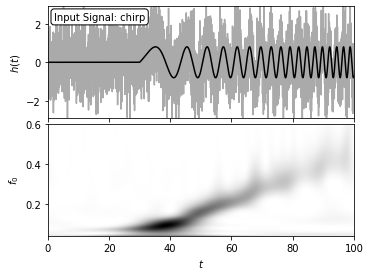# Temporally localized signals#

## Introduction#

A case frequently encountered in practice is a stationary signal with an event localized in time.
When the noise properties are understood, and the expected shape of the signal is known, a tool of choice is full forward modeling. That is, here too the analysis includes model selection and parameter estimation steps, and is often called a matched filter search.

In this notebook, we will discuss two simple parametric models:

1. a burst signal

2. a chirp signal

In both examples we assume Gaussian known errors. The generalization to nonparametric models and more complex models can be relatively easily implemented by modifying the code developed for these two examples.

## Import functions#

We use functions from PyMC3. We will also use FT_continuous, IFT_continuous, and wavelet_PSD from astroML.fourier for wavelet PSD analysis.

import numpy as np
from matplotlib import pyplot as plt

import pymc3 as pm

from astroML.plotting.mcmc import plot_mcmc

from astroML.fourier import FT_continuous, IFT_continuous, wavelet_PSD


# Matched Filter Chirp Search#

A more complex ten-parameter case of chirp modeling is show in this matched filter chirp search example. The chirp signal is temporally localized and it decays exponentially for t > T:

$y_C(t|T, A, \phi, \omega, \beta) = A sin[\phi + \omega(t - T) + \beta(t - T)^2] exp[-\alpha(t - T)]$

## 1. set up a chirp model example#

Using equation $$y(t) = b_0 + Asin[\omega t + \beta t^2])$$, we set up a chirp model as an example to show the matched filter search.
The chirp data set is shown in the plot below.

# Set up toy dataset
def chirp(t, T, A, phi, omega, beta):
"""chirp signal"""
signal = mask * (A * np.sin(phi + omega * (t - T) + beta * (t - T) ** 2))
return signal

def background(t, b0, b1, omega1, omega2):
"""background signal"""
return b0 + b1 * np.sin(omega1 * t) * np.sin(omega2 * t)

np.random.seed(0)
N = 500
T_true = 30
A_true = 0.8
phi_true = np.pi / 2
omega_true = 0.1
beta_true = 0.02
b0_true = 0.5
b1_true = 1.0
omega1_true = 0.3
omega2_true = 0.4
sigma = 0.1

t = 100 * np.random.random(N)

signal = chirp(t, T_true, A_true, phi_true, omega_true, beta_true)
bg = background(t, b0_true, b1_true, omega1_true, omega2_true)

y_true = signal + bg

y_obs = np.random.normal(y_true, sigma)

t_fit = np.linspace(0, 100, 1000)
y_fit = (chirp(t_fit, T_true, A_true, phi_true, omega_true, beta_true) +
background(t_fit, b0_true, b1_true, omega1_true, omega2_true))

# show the model
fig = plt.figure(figsize=(9, 9))
ax = fig.add_axes([0.52, 0.7, 0.43, 0.25])
ax.scatter(t, y_obs, s=9, lw=0, c='k')
ax.set_xlim(0, 100)
ax.set_xlabel('$t$')
ax.set_ylabel(r'$h_{\rm obs}$')

Text(0, 0.5, '$h_{\\rm obs}$')## 2. Set up and run the MCMC sampling#

We use MCMC to compute the posterior parameters in the equation, in order to fit this chirp model. The parameters we estimate using MCMC are $$T$$, $$A$$, $$\omega$$, and $$\beta$$.

# Set up and run MCMC sampling

with pm.Model():

T = pm.Uniform('T', 0, 100, testval=T_true)
A = pm.Uniform('A', 0, 100, testval=A_true)
phi = pm.Uniform('phi', -np.pi, np.pi, testval=phi_true)

b0 = pm.Uniform('b0', 0, 100, testval=b0_true)
b1 = pm.Uniform('b1', 0, 100, testval=b1_true)

log_omega1 = pm.Uniform('log_omega1', -3, 0, testval=np.log(omega1_true))
log_omega2 = pm.Uniform('log_omega2', -3, 0, testval=np.log(omega2_true))

omega = pm.Uniform('omega', 0.001, 1, testval=omega_true)
beta = pm.Uniform('beta', 0.001, 1, testval=beta_true)

y = pm.Normal('y', mu=(chirp(t, T, A, phi, omega, beta)
+ background(t, b0, b1, np.exp(log_omega1), np.exp(log_omega2))),
tau=sigma ** -2, observed=y_obs)

step = pm.Metropolis()

traces = pm.sample(draws=5000, tune=2000, step=step, return_inferencedata=False)

labels = ['$T$', '$A$', r'$\omega$', r'$\beta$']
limits = [(29.75, 30.25), (0.75, 0.83), (0.085, 0.115), (0.0197, 0.0202)]
true = [T_true, A_true, omega_true, beta_true]

Multiprocess sampling (4 chains in 4 jobs)
CompoundStep
>Metropolis: [beta]
>Metropolis: [omega]
>Metropolis: [log_omega2]
>Metropolis: [log_omega1]
>Metropolis: [b1]
>Metropolis: [b0]
>Metropolis: [phi]
>Metropolis: [A]
>Metropolis: [T]

100.00% [28000/28000 00:16<00:00 Sampling 4 chains, 0 divergences]
Sampling 4 chains for 2_000 tune and 5_000 draw iterations (8_000 + 20_000 draws total) took 25 seconds.
The rhat statistic is larger than 1.2 for some parameters.
The estimated number of effective samples is smaller than 200 for some parameters.


## 3. Show estimated model#

A matched filter search for a chirp signal in time series data.

• The top-right panel shows a simulated data set generated from the chirp model.

• The posterior pdf for the four model parameters ($$T$$, $$A$$, $$\omega$$, and $$\beta$$) is determined using MCMC and shown in the other panels.

• Seven of the parameters can be considered nuisance parameters, and we marginalize over them in the likelihood contours shown here.

# Plot results
fig = plt.figure(figsize=(9, 9))

# This function plots multiple panels with the traces
axes_list = plot_mcmc([traces[i] for i in ['T', 'A', 'omega', 'beta']],
labels=labels, limits=limits,
true_values=true, fig=fig,
bins=30, colors='k',
bounds=[0.14, 0.08, 0.95, 0.95])

for ax in axes_list:
for axis in [ax.xaxis, ax.yaxis]:
axis.set_major_locator(plt.MaxNLocator(5))

ax = fig.add_axes([0.52, 0.7, 0.43, 0.25])
ax.scatter(t, y_obs, s=9, lw=0, c='k')
ax.plot(t_fit, y_fit, '-k')
ax.set_xlim(0, 100)
ax.set_xlabel('$t$')
ax.set_ylabel(r'$h_{\rm obs}$')

Text(0, 0.5, '$h_{\\rm obs}$')## Chirp wavelet PSD#

The signal in the absence of chirp is taken as

$y(t) = b_0 + b_1 sin(\omega_1 t) sin(\omega_2 t)$

Here, we can consider parameters $$A$$, $$\omega$$, $$\beta$$, and $$\alpha$$ as “interesting,” and other parameters can be treated as “nuisance.”
We will take the same chirp signal above and draw a wavelet PSD for demonstration.

### 1. compute the wavelet PSD of chirp model#

We use the previous complex ten-parameter case of chirp modeling, and compute its wavelet PSD.

np.random.seed(42)
N = 4096
t = np.linspace(-50, 50, N)
h_true = chirp(t, -20, 0.8, 0, 0.2, 0.02)
h = h_true + np.random.normal(0, 1, N)

f0 = np.linspace(0.04, 0.6, 100)

# compute wavelet PSD
wPSD = wavelet_PSD(t, h, f0, Q=1.0)


### 2. plot signal and wavelet#

Here, the signal with an amplitude of A = 0.8 is sampled in 4096 evenly spaced bins, and with Gaussian noise with sigma = 1. The two-dimensional wavelet PSD easily recovers the increase of characteristic chirp frequency with time.

# Plot the  results
fig = plt.figure(figsize=(5, 3.75))

# Top: plot the data
ax.plot(t + 50, h, '-', c='#AAAAAA')
ax.plot(t + 50, h_true, '-k')

ax.text(0.02, 0.95, "Input Signal: chirp",
ha='left', va='top', transform=ax.transAxes,
bbox=dict(boxstyle='round', fc='w', ec='k'))

ax.set_xlim(0, 100)
ax.set_ylim(-2.9, 2.9)
ax.xaxis.set_major_formatter(plt.NullFormatter())
ax.set_ylabel('$h(t)$')

# Bottom: plot the 2D PSD
ax.imshow(wPSD, origin='lower', aspect='auto',
extent=[t + 50, t[-1] + 50, f0, f0[-1]],
cmap=plt.cm.binary)

ax.text(0.02, 0.95, ("Wavelet PSD"), color='w',
ha='left', va='top', transform=ax.transAxes)

ax.set_xlim(0, 100)
ax.set_ylim(0.04, 0.6001)
ax.set_xlabel('$t$')
ax.set_ylabel('$f_0$')

plt.show()